NSO Result Declared Check here | NSO Level 2 Exam Timing & Guidelines Check here | Register for Maths, Science, English, GK Olympiad Exam Click here | Check Olympiad Exam Dates here | Buy Practice Papers for IMO, IOM, HEO, IOEL etc here | Login here to participate in all India free mock test on every Saturday

# Light Sound and ForceWe see through our eyes, hear through our ears and push things through our hands. Does seeing, hearing and pushing have some science involved in it?

Let us first talk about light. We are able to see objects because of light but can you think how an object becomes visible to you?

When light hits an object some part of it bounces back and reaches our eyes. This is called reflection. Look at the picture given below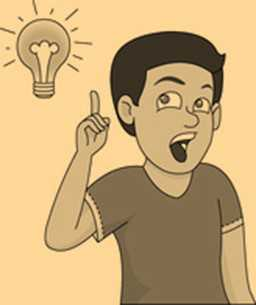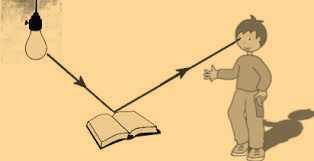The light that comes from the bulb and strikes the book is called incident ray denoted by the symbol I and the light that bounces back from the book and reaches the boy is called the reflected ray denoted by r. Now if you draw a perpendicular line that is a 90-degree line in between the incident and reflected ray, that line is known as normal. Looking at the diagram below will give you more idea.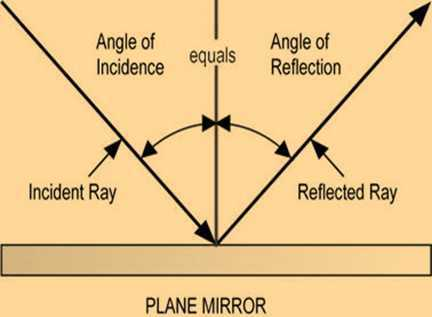The red line is normal and it makes a 90-degree angle with the plane mirror.

The angle that the incident ray makes with the normal is called angle of incidence denoted by ∠i.

The angle that is formed by normal and the reflected ray is called angle of reflection denoted by        ∠r. Remember that angle of incidence is always equal to the angle of reflection that is ∠i = ∠r.

Now let us talk about mirrors. Do you know that there are different types of mirrors as well? Ever noticed the mirror that is used in the cars? The mirror used in the cars is different from the mirror you use at home to see yourself.

There are basically three types of mirrors

• Plane mirrors that you use to see yourself at homes, washrooms etc. These are the normal and flat ones.
• Concave mirrors are used in car headlights, spotlights, telescopes etc. These are curved inwards like a spoon.
• Convex mirrors are used in cars. These are curved outwards unlike concave mirrors.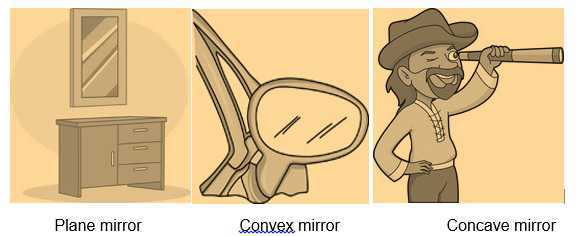• Enough of light lessons now let us move to sound.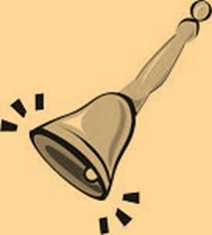So how do you know when a period is over in your school? By hearing the sound of the bell, right?

But how is the sound produced? The sound is produced whenever a body vibrates or moves to and fro.

So the to and fro or back and forth motion of an object is termed as vibration.

Try this fun experiment at home. Take two plastic cups and punch holes in both the cups. Now tie both the cups together with a long string. Do you know this can act as a telephone?

Also, this experiment proves that sound needs a medium to travel. Sound can travel through solid, liquid, glass or space. You will learn more about sound in higher classes. Let us move to force.Look at this picture, the lady is dragging her son to go to school. In short, the lady is applying force on the kid. So a normal push or pull is called force. You use force every day, while writing, playing, dancing etc. Do you know what else force can do?

A Force can Change the State of Motion

For example, while taking a penalty kick in football, the player applies a force on the ball. Before being hit, the ball was at rest and so its speed was zero. The applied force makes the ball move towards the goal.

Force can Change the Shape of an Object

What happens when you apply a force on an inflated balloon by pressing it between your palms? Think about it.

You have learned the basics of light, sound, and force.

Let’s recall some important keywords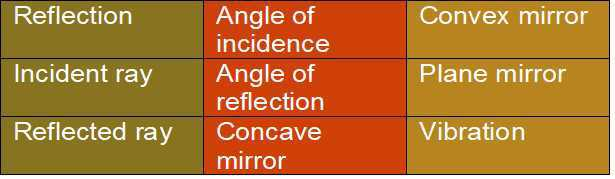## Quiz for Light Sound and Force

 Q.1 Fill in the blank. When something blocks the passage of light a ______ is formed a) Image b) Picture c) Shadow d) Force
 Q.2 Which of these can be applied to move a resting object? a) Water b) Wood c) Shadow d) Force
 Q.3 Fill in the following blank:- When we apply force to a chair and it _____ _, so we can say work is done. a) vanishes b) does not move c) moves d) none of the above
 Q.4 Which of these is not a source of energy? a) Atom b) Sun c) Glass d) Water
 Q.5 A is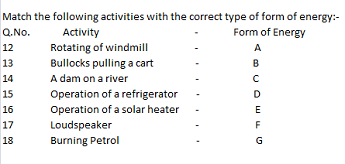a) Wind Energy b) Solar Energy c) Muscular Energy d) Water Energy
 Q.6 B isa) Wind Energy b) Solar Energy c) Muscular Energy d) Water Energy
 Q.7 C isa) Wind Energy b) Solar Energy c) Muscular Energy d) Water Energy
 Q.8 D isa) Solar Energy b) Electrical Energy c) Muscular Energy d) Water Energy
 Q.9 E isa) Solar Energy b) Electrical Energy c) Heat Energy d) Sound Energy
 Q.10 F isa) Solar Energy b) Electrical Energy c) Heat Energy d) Sound Energy